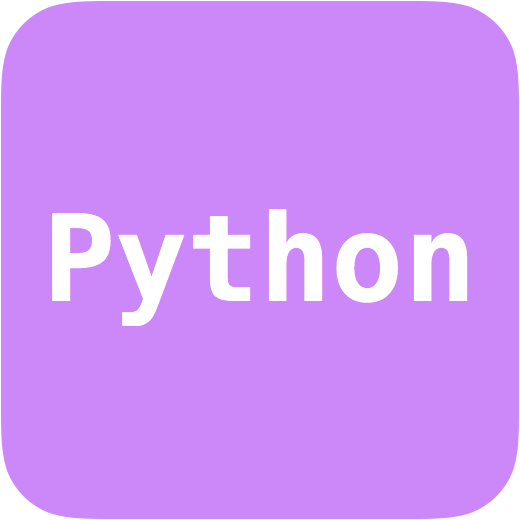# MGCNNAuthors: F. Lalande, A. Peel Language: Python 3 Download: mgcnn.tar.gz Description: A Convolutional Neural Network (CNN) architecture for classifying standard and modified gravity (MG) cosmological models based on the weak-lensing convergence maps they produce.

## Introduction

This repository contains the code and data used to produce the results in A. Peel et al. (2018), arXiv:1810.11030.

The Convolutional Neural Network (CNN) is implemented in Keras using TensorFlow as backend. Since the DUSTGRAIN-pathfinder simulations are not yet public, we are not able to include the original convergence maps obtained from the various cosmological runs. We do provide, however, the wavelet PDF datacubes derived for the four models as described in the paper: one standard LCDM and three modified gravity f(R) models.

## Requirements

• Python 3
• numpy
• Keras with Tensorflow as backend
• scikit-learn

## Usage

\$ python3 train_mgcnn.py -n0

The three options for the noise flag "-n" are (0, 1, 2), which correspond to noise standard deviations of sigma = (0, 0.35, 0.70) added to the original convergence maps. Additional options are "-i" and "-e" for the number of training iterations and epochs, respectively.

Confusion matrices and evaluation metrics (loss function and validation accuracy) are saved as numpy arrays in the generated output/ directory after each iteration.